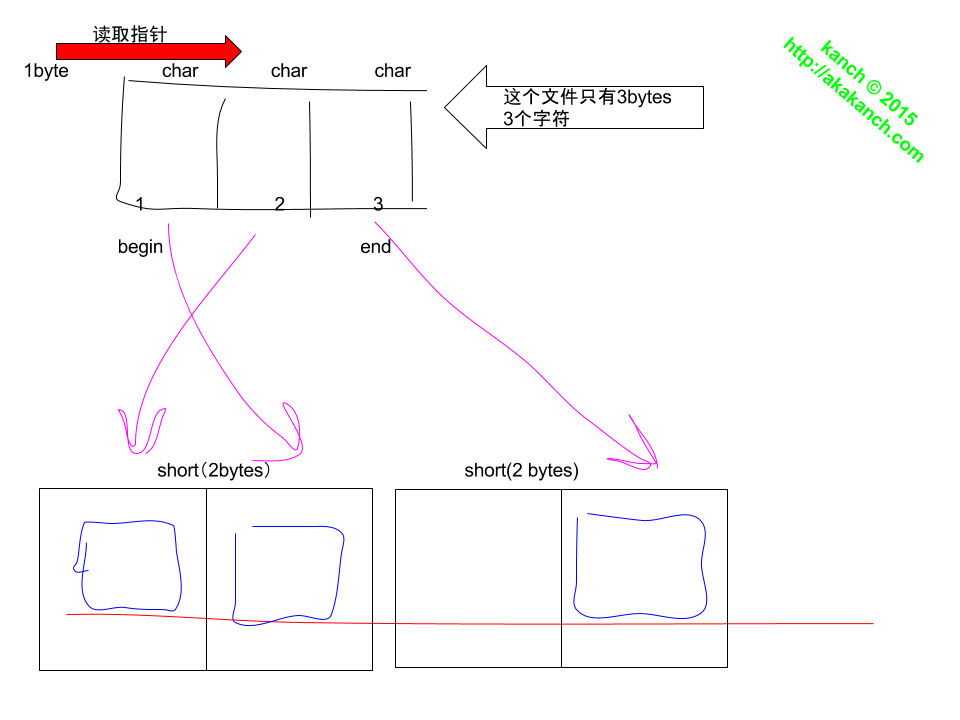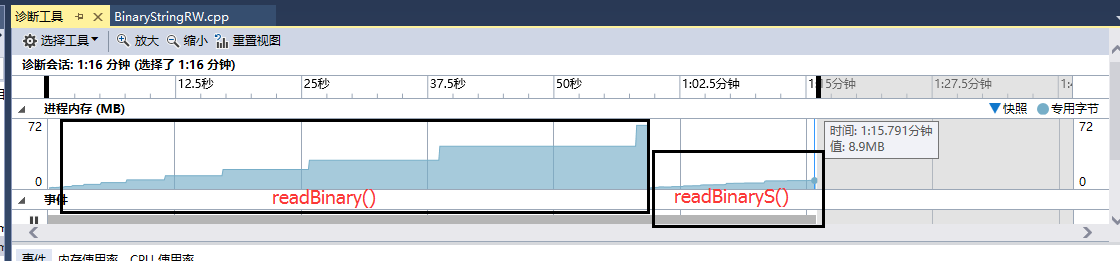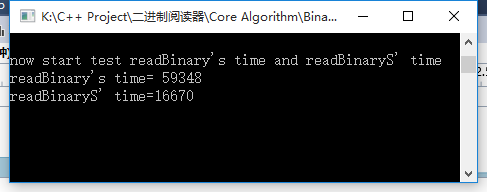# kanch.blog

posted at 2015-10-18 23:29:56 +0000

# C++实现将文件二进位制作为字符串读出与写入字符串形式的二进制数据

``````00010001011110001
``````

## 读取文件的二进制位

``````ifstream read(filepath, ios_base::binary | ios_base::in | ios_base::ate);  //open specfic file,then move pointer to the end of file
int fileylen = read.tellg();   //tell  the len of the file (bytes)
read.seekg(0, ios_base::beg);  //set pointer to the begining of the file
``````

``````short SV = { short(0x1),short(0x2),short(0x4),short(0x8),
short(0x10),short(0x20),short(0x40),short(0x80),
short(0x100),short(0x200),short(0x400),short(0x800),
short(0x1000),short(0x2000),short(0x4000),short(0x8000)
};
``````

``````for (int m = 0; m < sizeof(short); m++)
{
if ((sbuf & SV[m]) == SV[m])
{
binBuf += "1";
}
else
{
binBuf += "0";
}
}
``````

## 根据已有字符串形式的二进制数写入

``````for (int m = 0; m < sizeof(short); m++)
{
if (binBuf[m] == '1')   //now set the bit of short
{
sbuf = (sbuf | SV[m]);
}

}
write.write((char*)&sbuf, sizeof(short));
sbuf = 0;        //set all bits to 0
``````

## 读取顺序## 关于算法效率的问题**<转载请注明出处>**

`© kanch`zl AT kanchz DOT com

last updated on 2019-11-12 02:36:10 +0000